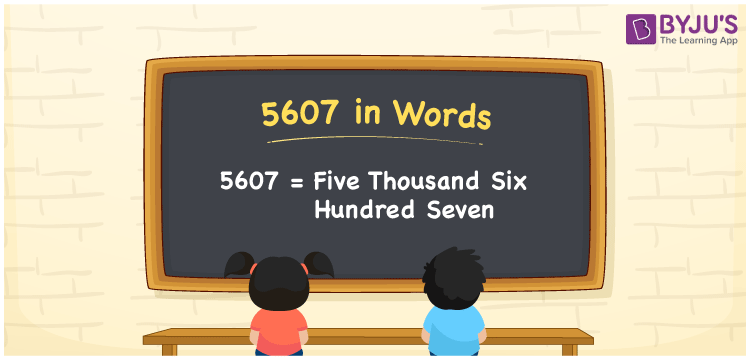# 5607 in Words

We can write 5607 in words as Five thousand six hundred seven. Thus, the word form or the number name of 5607 is given by Five thousand six hundred seven. This representation is useful whenever we are asked to spell or express the cardinal number 5607. For instance, Rs. 5607 can be spelt as “Five thousand six hundred seven rupees”. Similarly, we use the word form of numbers in many general conversations.

 5607 in words Five thousand six hundred seven Five thousand six hundred seven in Numbers 5607

## 5607 in English Words

We generally write numbers in words using the English alphabet. Thus, we spell 5607 in English words as “Five thousand six hundred seven”.## How to Write 5607 in Words?

To learn how to write the number 5607 in words, we need to make a place value chart with four columns since it has four digits. This can be done as follows.

 Thousands Hundreds Tens Ones 5 6 0 7

Here, ones = 7, tens = 0, hundreds = 6, and thousands = 5.

So, 5 × Thousand + 6 × Hundred + 0 × Ten + 7 × One

= 5 × 1000 + 6 × 100 + 0 × 10 + 7 × 1

= 5000 + 600 + 7

= Five thousand + Six hundred + Seven

= Five thousand six hundred seven

Therefore, 5607 in words = Five thousand six hundred seven.

Also, check: place value

5607 is a natural number that precedes 5608 and succeeds 5606.

5607 in words – Five thousand six hundred seven

Is 5607 an even number? – No

Is 5607 an odd number? – Yes

Is 5607 a perfect square number? – No

Is 5607 a perfect cube number? – No

Is 5607 a prime number? – No

Is 5607 a composite number? – Yes

## Frequently Asked Questions on 5607 in Words

Q1

### How do you write 5607 in words?

We can write the number 5607 in words as Five thousand six hundred seven.
Q2

### How to write an amount Rs. 5607 in words on a cheque?

On a cheque, we generally write an amount of Rs. 5607 in words as “Five thousand six hundred seven rupees only”.
Q3

### Write 5607.85 in words.

The number 5607.85 can be written in words as “Five thousand six hundred seven point eight five”.